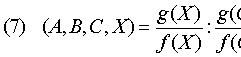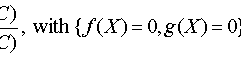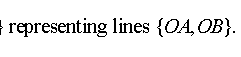##1. Projective Line

The standard model of the projective line consists of the classes X= [x] = [x1, x2] of points of R2-{(0,0)} modulo non-zero multiplicative constants. For every non-zero vector a of R2, A=[a] denotes a point of the projective line and a is called a representative of the point. Two representatives a, a' defining the same point if and only if a' = ka, with a non-zero real number k.

The most important examples of projective lines are the usual lines of the euclidean plane to which we add an additional point, called point at infinity. This additional point makes the line closed and is called projectification of the line (or one-point-compactification). The projectification is illustrated by the following picture: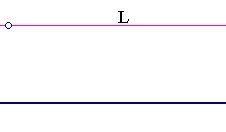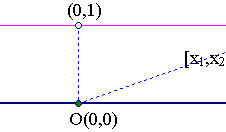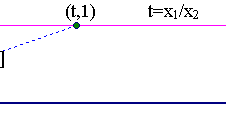Consider the points of line L: y=1, parallel to the x-axis. Each line represented by [x1,x2], with non-zero x2, intersects L at the point (t,1) with t=x1/x2. The line parallel to L which is the x-axis represented by [x1,0] adds an additional point to L, considered as the intersection point (at infinity) of the two parallel lines.

The set A* of all euclidean lines of the plane passing through the point A generalizes the basic model of projective line. Often A* is called the pencil of lines through A.

##2. Homogeneous projective coordinates

The pairs (x1,x2) defining line [x1,x2] of the projective line are called homogeneous projective coordinates. They are defined modulo a multiplicative constant, since (kx1,kx2) defines the same point. Also they cover all the points of the projective line except a single one.
Obviously another system (x'1,x'2) of coordinates of R2 (with the same origin) is related to (x1,x2) by an invertible matrix: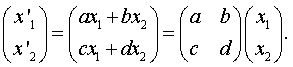And for the corresponding quotients t'=x'1/x'2, t=x1/x2 we get the homographic relation: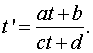##3. Projective base

Three distinguished points {A,B,C} on a projective line determine a so-called projective base and through it a corresponding homogeneous system of coordinates. The work is done in terms of bases of the vector space R2.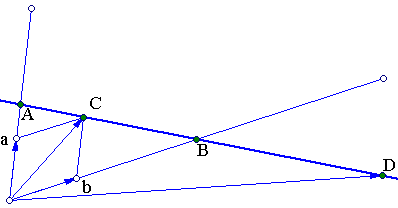The points determine three lines represented by corresponding vectors {a,b,c}: A=[a], B=[b], C=[c]. The vectors are selected so that c=a+b. This condition uniquely determines {a,b,c} up to a multiplicative constant. The homogeneous coordinate system results by using the basis {a,b} and writing vor every point D on this line its coordinates with respect to this basis D=[d], where d=d1a+d2b. Thus to D we correspond (d1,d2).
Obviously in this coordinate system {A,B,C} have correspondingly the coordinates {(1,0), (0,1), (1,1)}.
C is called coordinator or unit of the projective base {A,B,C}. It is used to calibrate a basis defined by the other two points. The calibration is done by projecting some c, such that C=[c] parallel to the other lines determined by {A,B}.

##4. Line projectivities

A bijective map F: M --> N between two projective lines {M,N} is called a projectivity, when its representation in homogeneous coordinates is a linear map.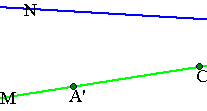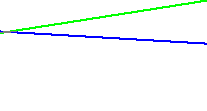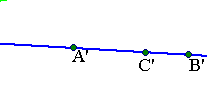This means that selecting any projective bases {A,B,C} on M and {A',B',C'} on N and using the corresponding coordinate systems (x,y) and (x',y'), the transformation Q=F(P) is described in the respective homogeneous coordinates through linear equations: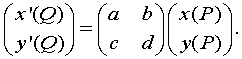Since the change of homogeneous coordinates in each projective line is done also through linear maps, a change of homogeneous coordinates replaces the above matrix U with a matrix U'=XUY, where {X,Y} are also invertible matrices related to the coordinate changes in M and N.

##5. Line perspectivities

The most prominent example of projectivity is the line perspectivity by which points on two projective lines {M,N} correspond through a map Q=F(P), such that line PQ passes through a fixed point R, called the center of perspectivity.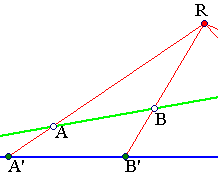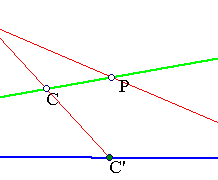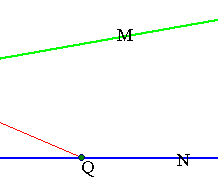The image above illustrates such a map. To verify the defining condition we take R to be the origin of coordinates and the projective bases {A,B,C} on M and {A'=F(A),B'=F(B),C'=F(C)} on N. With respect to this bases map F is described through the identity transformation (x'(Q),y'(Q)) = (x(P),y(P)), which is the simplest invertible linear map.
File RectHypeRelation.html discusses the representation of such a perspectivity referred to more general projective bases on the lines M and N.

##6. Cross ratio

To define the cross-ratio (or anharmonic ratio) of four points {P,Q,U,V} on a projective line one can again use homogeneous coordinates and write {(x1(P),y1(P)), (x2(Q),y2(Q)), (x3(U),y3(U)), (x4(V),y4(V))}. Then define the corresponding quotients {p=x1/y1, q=x2/y2, u=x3/y3, v=x4/y4}. Finally define the cross ratio through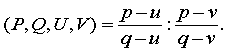The important point is again that this definition, although it uses homogeneous coordinates, it defines a number independent of the particular coordinates used. In fact, changing to another system of homogeneous coordinates, according to (2) changes {p,q,u,v} to {p',q',u',v'} such that: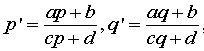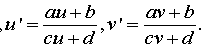In other words {p,q,u,v} and {p',q',u',v'} are related through a homographic relation and the clue is that this relation preserves the value of the cross ratio (this needs a short calculation) i.e.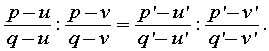Remark-1 The same reason i.e. the preservation of cross ratio by homographic relations implies that line-projectivities preserve also the cross ratio. This means that for a projectivity F : M --> N between two projective lines and for any four points in general position (no coincidences) {P,Q,U,V} and their images {P'=F(P),Q'=F(Q),U'=F(U),V'=F(V)} we have the equality: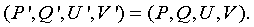Remark-2 Taking {P,Q} as the first points of projective base, so that in the corresponding coordinate system these points have coordinates {(1,0) , (0,1)}, we get p=inf (inf denoting "infinity") and q=0, and the cross ratio reduces to: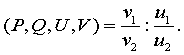Here (u1,u2) and (v1,v2) denote the coordinates correspondingly of {U,V} with respect to this particular coordinate system.

The ubiquitous homographic relation is studied in some detail in the file HomographicRelation.html .

##7. Cross ratio and length measurements

The cross ratio, as we saw, is independent of any metric measurements. For lines of the euclidean plane though we can use distances and calculate the cross ratio from various measurements related to the four points {A,B,C,D} on a line. The figure below illustrates the connexion between the metric-independent definition and the calculations using distances.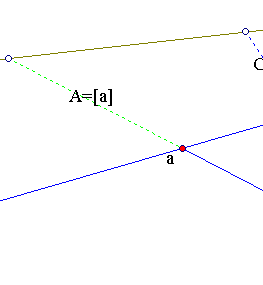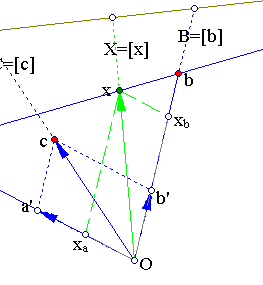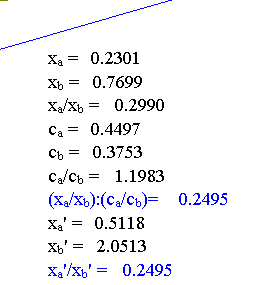The figure shows how the coordinates of the four points {[a],[b],[c],[x]} depend on the particular vectors {a,b,c,x} representing these points. Vector x is decomposed with respect to {a',b'}, which are determined by the (parallel) projections {a',b'} of c on {a,b} and not by {a,b} themselves. This shows how the coordinator vector c calibrates the basis vectors (leading to {a',b'}). Thus we write, x = xa'a'+xb'b', giving the equation: [x] = xa'[a] + xb'[b], expressing X = xa'A+xb'B. The pair (xa',xb') gives the projective coordinates of point X with respect to the projective base (of the line) determined by the tripple of points {A=[a'], B=[b'], C=[c]}.
Number d = xa'/xb' is the same with the cross ratio (A,B,C,X)=(xa/xb):(ca/cb), where the coordinates (xa,xb) and (ca,cb) are taken with respect to {a,b} or any other system {ra,sb} of (non-zero) multiples of {a,b}.

Remark-1 Ratio xa/xb can be expressed through the ratio of euclidean signed distances d(x,a)/d(x,b). The relation follows by applying the sine theorem to triangles axax and xxbb (both similar to aOb), by which, d(x,a)=pxb and d(x,b)=qxa, where {p,q} depend on the fixed triangle aOb. Thus d(x,a)/d(x,b) = (xb/xa)(p/q).

Remark-2 Distances {d(x,a), d(x,b)} can be expressed also through the orthogonal distances {d(X,[a]),d(X,[b])} of X from lines {a,b} respectively. In fact, d(X,[a])=r*d(x,a), d(X,[b])=s*d(x,b), where {r,s} denote the sines of the angles at {a,b}. Thus
d(X,[a]):d(X,[b]) = (d(x,a)/d(x,b))*(r/s) = (xb/xa)*(p/q)*(r/s).
In total we have the following possibilities to calculate the cross ratio: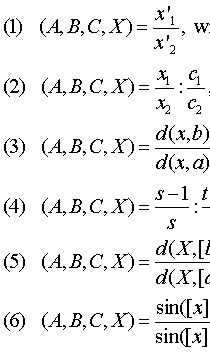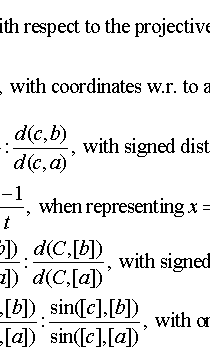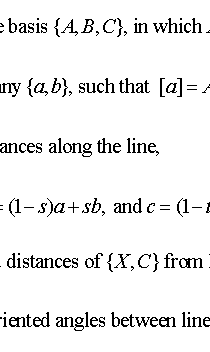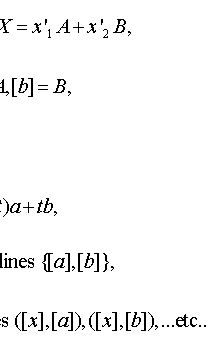Last expression follows from (5) by dividing both members of the ratio d(X,[b])/d(X,[a]) with distance d(O,X) and both members of ratio d(C,[b])/d(C,[a]) with distance d(O,C). In the last two expressions points {A,B,C,X} are identified with points on the supporting euclidean line.

##8. Cross ratio and line equations

Assume that lines OA, OB are given by equations correspondingly {f(x,y)=0, g(x,y)=0}. Where f(x,y)=px+qy+r and g(x,y)=p'x+q'y+r'. For any other point P=(p1,p2) of the plane denote by f(P) the number f(p1,p2), which is a multiple of the distance of P from the line f(x,y)=0. Thus, g(X)/f(X) = k*(d(X,[b])/d(X,[a])), k being a constant independent of X. This leads to another simple expression of the cross ratio involving the functions {f,g} expressing lines {OA,OB}: